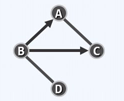DSA学习笔记

学习一个

Posted by cj on March 22, 2019

DSA学习笔记

二叉树

Quiz

1. In the graph implemented with adjacency matrices with n vertices, the vertex v has m neighbors, and the time complexity of traversing all m neighbors is: 在包含n个顶点的用邻接矩阵实现的图中，顶点v有m个邻居，遍历所有m个邻居的时间复杂度为：

A. O(1); B. O(m); C. O(n); D. O(mn);

因为是邻接矩阵实现，空间为O(n*n), 扫描v的邻居需要扫描一行即 O(n)次

2. G is a directed acyclic graph, and (u, v) is an edge in G that points from u to v. The result of DFS on G is: G是有向无环图，(u, v)是G中的一条由u指向v的边。对G进行DFS的结果是：

A. dTime(u) > dTime(v); B. dTime(u) < dTime(v); C. fTime(u) > fTime(v); D. fTime(u) < fTime(v);

有向且无环，无法推出B，因为还可能存在其他指向v的边。只能推出 C. fTime(u) > fTime(v)，因为无论如何，必然是v先完成访问达到visited状态。

图

Quiz

1. Q: In a simple undigraph with 20 vertices, the maximum number of edges is ? 在含20个顶点的简单无向图中，边的数量最多为? The degree of the vertex with the smallest degree at this time is ? 此时度最小的顶点的度为 ?

A: 当n阶简单无向图为完全图时，任意节点都与其余n-1个节点邻接，边的数量最多，为 n(n-1)/2。因此，当n=20时，边数最多为190；每个顶点都与其余顶点邻接，所有顶点的度数都是19。

2. Q: A total of 7 people took part in the banquet and a friendly handshake took place among the participants. The number of hands-on handshakes known to each of them is:

3, 1, 2, 2, 3, 1, 2

How many handshakes have occurred at the banquet?

某宴会一共有7个人参加，与会者之间进行了亲切的握手。已知他们中的每个人进行握手的次数分别为：

3, 1, 2, 2, 3, 1, 2

请问宴会上总共发生了多少次握手？

In the long history of humanity, everyone may have to shake hands with other people. If someone makes an odd number of handshakes in his life, he is called a Class A person, otherwise he is called a Class B person. The number of people of type A since ancient times is: (assuming that humans can only shake hands with humans)

在人类的历史长河中，每个人都可能要与其他人握手。如果某人在他的一生中进行握手的次数为奇数，则称他为A类人，否则称为B类人。试问从古至今A类人的个数是：(假设人类只能和人类握手)

A. Even number 偶数 B. Odd number 奇数

C. Prime number 素数 D. Complete square number 完全平方数

A: 7, A。 (3+1+2+2+3+1+2)/2 = 7。 具体可参考这篇握手定理理解，讲的比较通俗易懂。

3. Q:The adjacency matrix of the above digraph is (in order of A, B, C, D) 以上有向图的邻接矩阵为（图中顶点以A、B、C、D为顺序）

A: 画一下就知道了

0 A B C D
A 0 0 1 0
B 1 0 1 1
C 1 0 0 0
D 0 1 0 0

搜索树

BST 二叉搜索树

• 中序遍历序列单调非降
• 平均高度
• 随机生成 randomly generated 时，平均高度θ(logn)
• 随机组成 randomly composed 时，平均高度θ(sqrt(n))（根号下n）
• 查找
• 取决于该节点的深度，或树的高度，O(h)
• 最好：目标出现在树根（或附近），O(1)
• 最坏：BST退化成单链，O(n)
• 插入
• 过程：搜索、插入、更新树高
• 复杂度：同查找，O(h)
• 删除
• 过程：搜索、删除、更新树高
• 复杂度：同查找于插入，O(h)
• 综述：查找、插入、删除等主要操作的效率均线性正比于BST的高度，而在最坏情况下，BST可能退化为列表，效率甚至降至O(n)

AVL树

• 由 G. M. Adelson-Velsky 和 E. M. Landis 于1962年发明，并以他们姓氏首字母命名
• 理想平衡：完全二叉树，过于苛刻
• 适度平衡：树高渐进地不超过O(logn)
• 查找：O(logn)
• 各节点平衡因子的绝对值均不超过1
• 高度为h的AVL树至少包含fib(h+3)-1个节点；包含n个节点的AVL树的高度应为O(logn)
• 失衡节点集：因节点x的插入或删除而暂时失衡的节点，构成失衡节点集，记作UT(x)
• 插入
• UT(x)可能包含多个节点
• 恢复平衡仅需不超过两次旋转，O(1)
• 复杂度：按照BST常规插入算法，需O(logn)；复衡需O(1)；整体为O(logn)
• 删除
• UT(x)仅含单个节点
• 恢复平衡可能需要O(h)次上溯，因此为O(logn)
• 复杂度：删除需先查找，O(logn)；复衡需O(logn)；整体O(logn)

Splay Tree 伸展树

• 是平衡二叉树，但无需时刻严格保持平衡
• 最坏情况复杂度与BST/AVL等相同Ω(n)，但分摊意义下在O(logn)以内
• 双层伸展
• 将被访问节点伸展至树根
• 使对应分支的长度以大致折半的速度收缩
• 不能杜绝最坏情况（访问最底层节点），但可以有效控制最坏情况发生的频率，从而在分摊意义下保证整体的高效率：O(logn)

B-树

• 由 R. Bayer 和 E. McCreight 于1970年合作发明（为毛不叫 BM Tree …）
• 多路搜索树(multi-way search tree)，充分利用内存的高速度与外存的大容量
• 多路平衡
• 所有外部节点深度相等
• m阶B-树(m>=2)，设s=(m/2)的上整，每个内部节点有[s, m]个分支，[s-1, m-1]个关键码
• 高度h=θ(log(m, N))，N为关键码总数
• 复杂度：查找、插入、删除均为O(log(m, N))，虽然没有渐进意义上的改进，但耗时的I/O操作的次数已经大致缩减为原先的1/log(2,m)。

RBTree 红黑树

• 每次插入或删除操作后的重平衡过程中，全树拓扑结构的更新仅涉及常数个节点
• 进一步放宽“适度平衡”的标准：任一节点左右子树的高度，相差不得超过两倍
• 红黑树的节点满足以下条件
1. 树根始终为黑色
2. 外部节点均为黑色
3. 其余节点若为红色，则其孩子节点必为黑色
4. 从任一外部节点到根节点的沿途，黑节点的数目相等
• 适当转换后，红黑树与4阶B-树等价
• 高度：可以控制在最小高度（完全二叉树高度O(logn)）的两倍以内，且从渐进的角度看仍是O(logn)，保证了适度平衡
• 查找：红黑树基于BST，O(logn)
• 插入：O(logn)
• 删除：O(logn)

Quiz

1. What’s the worst-case time complexity for searching in a BST with n nodes? 在含n个节点的BST中进行查找的最坏时间复杂度为：

A. O(1); B. O(log2(n)); C. O(n); D. O(nlog2(n));

最坏情况就是退化成单链的情况，此时查找的复杂度为O(n)。

2. Consider inserting a target element e into a BST. when it fails to be found during the search, which node does _hot correspond to? 对BST进行插入操作，对待插入的目标元素e进行查找后，若查找失败，_hot指向的节点为：

A. The node to be inserted 待插入的节点; B. The parent of e after the insertion e被插入后的父亲; C. The left-child of e after the insertion e被插入后的左孩子; D. The root 根节点

代码

static NodePtr& searchIn(NodePtr& v, const T& e, NodePtr & hot) {
if (!v || (e == v->data_)) { return v; }
hot = v;
return searchIn(((e < v->data_) ? v->lChild_ : v->rChild_), e, hot);
}

//! 查找
virtual NodePtr& search(const T& e) { return searchIn(this->root_, e, hot_ = nullptr); }

语义约定：

若查找成功，searchIn()search()的返回值指向一个关键码为e且真实存在的节点；若查找失败，则返回的数值虽然为nullptr，但它是一个引用，指向最后一次试图转向的节点（可以用作插入）。 在整个查找过程中，hot变量始终指向当前节点的父亲。因此算法返回时，_hot亦将统一指向“命中节点”的父亲。

因此对于本题，答案应为B。

3. When atempting to delete a node v of degree 2, what’s the actual node being deleted? 当欲删除的节点v在BST中的度为2时，实际被删除的节点为：

A. v’s direct predecessor in the in-order sequence v在中序遍历下的直接前驱; B. v’s direct successor in the pre-order sequence v在先序遍历下的直接后继; C. the last node in the left branch of v’s right sub-tree v的右子树中左侧分支的最后一个节点; D. v’s parent v的父亲;

节点v的度为2，即有两条边，分3种情况：

1. 节点v不是根节点，有一个父亲一个右孩子
2. 节点v不是根节点，有一个父亲一个左孩子
3. 节点v为根节点，有两个孩子

继续看代码

static NodePtr removeAt(NodePtr& x, NodePtr& hot) {
NodePtr w = x; // 实际被摘除的节点，初值同x
NodePtr succ = nullptr; // 实际被摘除的节点的接替者
if (!x->lChild) { // 左子树为空
succ = x = x->rChild_; // 接替者为其右子树（可能为空）
} else if (!x->rChild) { // 右子树为空
succ = x = x->lChild_; // 接替者为其左孩子（可能为空）
} else { // 左右子树并存的情况
w = w->succ(); std::swap(x->data_, w->data_); // 令x与其后继w互换数据
auto u = w->parent_;
(u == x ? u->rChild_ : u->lChild_) = succ = w->rChild_;
}

hot = w->parent_; // 记录实际被删除节点父亲
if (succ) { succ->parent_ = hot; } // 将被删除节点的接替者与hot相联
// todo 释放被摘除的节点 release(w->data); release(w);
return succ; // 返回接替者
}

//! 删除
virtual bool remove(const T& e) {
auto& x = search(e);
if (!x) { return false; }
removeAt(x, hot_);
this->size_--;
updateHeightAbove(x);
return true;
}

对于情况1和2，实际被删除的都是自身，注意参数x为引用类型，因此语义为将被摘除节点替换为其孩子节点。 对于情况3，删除的是v的右子树中左侧分支的最后一个节点。

这道题有点疑惑，按道理，对于情况1和2，并不能选C，但正确答案就是C，难道题目中的度数指的是出度？

4. What is the maximum number of imbalanced nodes after inserting a node in an AVL tree? 在AVL树中刚插入一个节点后失衡节点个数最多为

O(lgn)

What is the maximum number of imbalanced nodes after deleting a node in an AVL tree? 在AVL树中刚删除一个节点后失衡节点个数最多为

O(1)

5. What’s the number of distinct BSTs containing nodes {1, 2, 3 ,4}? 包含节点{1,2,3,4}的不同二叉搜索树有多少棵？（Hint 提示：recursion 递归）

设 f(n) 为 n 个互不相同的节点组成的、拓扑互异的 BST 数量。则：

明显 f(1) = 1;

n=2时， 分别选取两个节点作为树根，由于 BST 需满足中序遍历有序的特性，有两种，即 f(2) = f(1) + f(1);

n=3时，分别选取最小和最大的两个节点作为树根，各有 f(2) 种；选取中间节点作为树根时，分别考虑左子树和右子树的所有情况，显然都是 f(1)；即 f(3) = f(2) * 2 + f(1) * f(1);

n=4时，分别选取4个节点作为根节点，则 f(4) = f(3) + f(1) * f(2) + f(2) * f(1) + f(3);

n=5时，分别选取5个节点作为根节点，则 f(5) = f(4) + f(1) * f(3) + f(2) * f(2) + f(3) * f(1) + f(4);

类推，得到 f(n) = f(n-1) + f(1) * f(n-2) + … + f(n-2) * f(1) + f(n-1);

f(4) = 14;